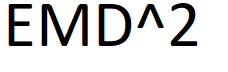#EMDD.KtGeometry 1.0.0

.NET 5.0
`Install-Package EMDD.KtGeometry -Version 1.0.0`
`dotnet add package EMDD.KtGeometry --version 1.0.0`
`<PackageReference Include="EMDD.KtGeometry" Version="1.0.0" />`
For projects that support PackageReference, copy this XML node into the project file to reference the package.
`paket add EMDD.KtGeometry --version 1.0.0`
`#r "nuget: EMDD.KtGeometry, 1.0.0"`
#r directive can be used in F# Interactive, C# scripting and .NET Interactive. Copy this into the interactive tool or source code of the script to reference the package.
```// Install EMDD.KtGeometry as a Cake Addin

// Install EMDD.KtGeometry as a Cake Tool
#tool nuget:?package=EMDD.KtGeometry&version=1.0.0```

## EMDD.KtGeometry

a library implementation of geometrical objects

### Requirements/Dependencies

.Net 5.0.102 sdk or greater

EMDD.KtCircularStack

### Nuget Package Usage

https://www.nuget.org/packages/EMDD.KtGeometry/

`<PackageReference Include="EMDD.KtGeometry" Version="1.*.*" />`

### Usage

• this library can be used to represent geometrical objects such as lines and plane as class type.

#### Vectors

• Vectors can be 2D or 3D through `EMDD.KtGeometry.KtVectors.KtVector2D` and `EMDD.KtGeometry.KtVectors.KtVector3D` respectively.

#### Points

• Points can be 2D or 3D through `EMDD.KtGeometry.KtPoints.KtPoint2D` and `EMDD.KtGeometry.KtPoints.KtPoint3D` respectively.

#### Lines

• Lines can be of type `KtLine2D`, `KtRay2D`, `KtSegment2D`, or `KtLine3D`
``````var line = new EMDD.KtGeometry.KtLines._2D.KtLine2D(2,3,-12, 3);

var startPoint = new EMDD.KtGeometry.KtPoints.KtPoint2D(2,3);
var direction = new EMDD.KtGeometry.KtVectors.KtVector2D(-1,4);
var endPoint = new EMDD.KtGeometry.KtPoints.KtPoint2D(5,5);

var ray = new EMDD.KtGeometry.KtLines._2D.KtRay2D(startPoint, direction);
var segment = new EMDD.KtGeometry.KtLines._2D.KtSegment2D(startPoint, endPoint);
``````
• Line methods includes line properties such as angle, intersepts and calculation of intersection points, distance from a point

#### KtPlane

• plane object
``````var plane = new EMDD.KtGeometry.KtPlanes.KtPlane(2,3,-12, 3); //2x + 3y - 12z = 3
``````
• able to calculate intesection with plane and line

#### KtPolygon

• polygons bound by edges
``````// a polygon contains a list of regions, where these regions can be +(addition/solid) or -(deductive/hollow)
var startPoint = new EMDD.KtGeometry.KtPoints.KtPoint2D(2,3);
var rectRegion = EMDD.KtGeometry.KtPolygons.CreateSpecialRegionMethods.Rectangle(startPoint, 20, 40, true);

var plane = new EMDD.KtGeometry.KtPolygons.KtPolygon2D(rectRegion);
``````
• Polygon method includes:
• Area calculation
• Polygon clipping
• Translation/rotation

#### Quaternions

• representation of graphical rotation and orientation of objects
``````var quatern= new KtQuaternion(w, x, y,z);
``````

#### todo

• better documentation
Product Versions
.NET net5.0 net5.0-windows net6.0 net6.0-android net6.0-ios net6.0-maccatalyst net6.0-macos net6.0-tvos net6.0-windows
Compatible target framework(s)